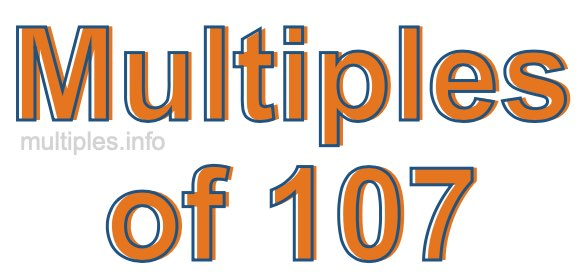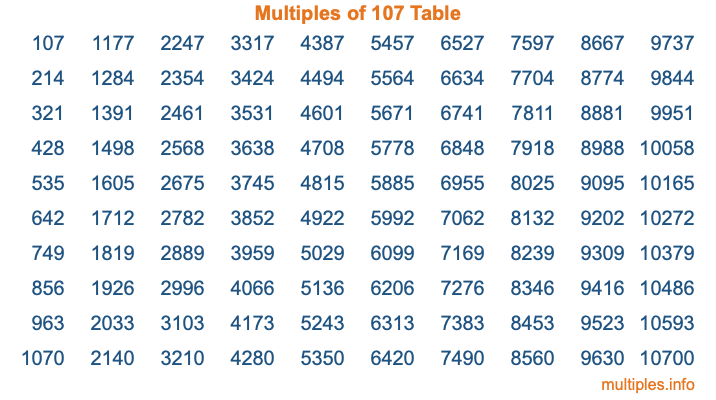Multiples of 107Welcome to the Multiples of 107 page. Here we will first teach you everything you will ever need to know about the multiples of 107, and then give you a study guide summary of everything we taught you to make sure you remember it all. Use this page to look up facts and learn information about the multiples of 107. This page will make you a multiples of one hundred seven expert!

Definition of Multiples of 107
Multiples of 107 are all the numbers that when divided by 107 equal an integer. Each of the multiples of 107 are called a multiple. A multiple of 107 is created by multiplying 107 by an integer.

Therefore, to create a list of multiples of 107, you start with 1 multiplied by 107, then 2 multiplied by 107, then 3 multiplied by 107, and so on for as long as you want. Thus, the list of the first five multiples of 107 is 107, 214, 321, 428, and 535. To see a larger list of multiples of 107, see the printable image of Multiples of 107 further down on this page. We also have a category where you can choose any nth multiple of 107.

Multiples of 107 Checker
The Multiples of 107 Checker below checks to see if any number of your choice is a multiple of 107. In other words, it checks to see if there is any number (integer) that when multiplied by 107 will equal your number. To do that, we divide your number by 107. If the the quotient is an integer, then your number is a multiple of 107.

Is  a multiple of 107?

Least Common Multiple of 107 and ...
A Least Common Multiple (LCM) is the lowest multiple that two or more numbers have in common. This is also called the smallest common multiple or lowest common multiple and is useful to know when you are adding our subtracting fractions. Enter one or more numbers below (107 is already entered) to find the LCM.

Check out our LCM Calculator if you need more details about the Least Common Multiple or if you need the LCM for different numbers for adding and subtraction fractions.

nth Multiple of 107
As we stated above, 107 is the first multiple of 107, 214 is the second multiple of 107, 321 is the third multiple of 107, and so on. Enter a number below to find the nth multiple of 107.

th multiple of 107

Multiples of 107 vs Factors of 107
107 is a multiple of 107 and a factor of 107, but that is where the similarities end. All postive multiples of 107 are 107 or greater than 107. All positive factors of 107 are 107 or less than 107.

Below is the beginning list of multiples of 107 and the factors of 107 so you can compare:

Multiples of 107: 107, 214, 321, 428, 535, etc.

Factors of 107: 1, 107

As you can see, the multiples of 107 are all the numbers that you can divide by 107 to get a whole number. The factors of 107, on the other hand, are all the whole numbers that you can multiply by another whole number to get 107.

It's also interesting to note that if a number (x) is a factor of 107, then 107 will also be a multiple of that number (x).

Multiples of 107 vs Divisors of 107
The divisors of 107 are all the integers that 107 can be divided by evenly. Below is a list of the divisors of 107.

Divisors of 107: 1, 107

The interesting thing to note here is that if you take any multiple of 107 and divide it by a divisor of 107, you will see that the quotient is an integer.

Multiples of 107 Table
Below is an image of the first 100 multiples of 107 in a table. The table is in chronological order, column by column. The first column has the first ten multiples of 107, the second column has the next ten multiples of 107, and so on.The Multiples of 107 Table is also referred to as the 107 Times Table or Times Table of 107. You are welcome to print out our table for your studies.

Negative Multiples of 107
Although not often discussed or needed in math, it is worth mentioning that you can make a list of negative multiples of 107 by multiplying 107 by -1, then by -2, then by -3, and so on, to get the following list of negative multiples of 107:

-107, -214, -321, -428, -535, etc.

Multiples of 107 Summary
Below is a summary of important Multiples of 107 facts that we have discussed on this page. To retain the knowledge on this page, we recommend that you read through the summary and explain to yourself or a study partner why they hold true.

There are an infinite number of multiples of 107.

A multiple of 107 divided by 107 will equal a whole number.

107 divided by a factor of 107 equals a divisor of 107.

The nth multiple of 107 is n times 107.

The largest factor of 107 is equal to the first positive multiple of 107.

107 is a multiple of every factor of 107.

107 is a multiple of 107.

A multiple of 107 divided by a divisor of 107 equals an integer.

107 divided by a divisor of 107 equals a factor of 107.

Any integer times 107 will equal a multiple of 107.

Multiples of a Number
Here you can get the multiples of another number, all with the same attention to detail as we did for multiples of 107 on this page.

Multiples of
Multiples of 108
Did you find our page about multiples of one hundred seven educational? Do you want more knowledge? Check out the multiples of the next number on our list!